# Ratio and Proportion - Quantitative Aptitude (MCQ) questions

Dear Readers, Welcome to Quantitative Aptitude Ratio and Proportion questions and answers with explanation. These Ratio and Proportion solved examples with shortcuts and tricks will help you learn and practice for your Placement Test and competitive exams like Bank PO, IBPS PO, SBI PO, RRB PO, RBI Assistant, LIC,SSC, MBA - MAT, XAT, CAT, NMAT, UPSC, NET etc.

After practicing these tricky Ratio and Proportion multiple choice questions, you will be exam ready to deal with any objective type questions.

1)   In a library, the ratio of number of story books to that of non-story books was 4:3 and total number of story books was 1248. When some more story books were bought, the ratio became 5:3. Find the number of story books bought.
- Published on 08 May 17

a. 312
b. 321
c. 936
d. 1560
 Answer  Explanation ANSWER: 312 Explanation: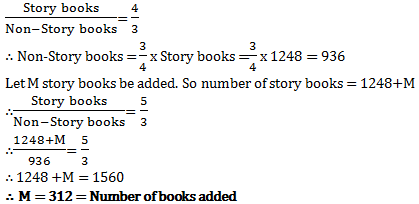2)   Rs. 8400 is divided among A, B, C and D in such a way that the shares of A and B, B and C, and C and D are in the ratios of 2:3, 4:5 and 6:7 respectively. The share of A is
- Published on 11 Apr 17

a. Rs. 1280
b. Rs. 8400
c. Rs. 8210
d. Rs. 1320
 Answer  Explanation ANSWER: Rs. 1280 Explanation: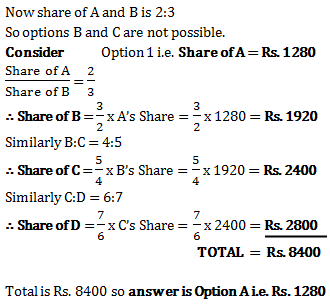3)   The ratio of the present age of father to that of son is 7:2. After 10 years their ages will be in the ratio of 9:4. The present ages of the father is
- Published on 11 Apr 17

a. 35 years
b. 40 years
c. 30 years
d. 25 years
 Answer  Explanation ANSWER: 35 years Explanation: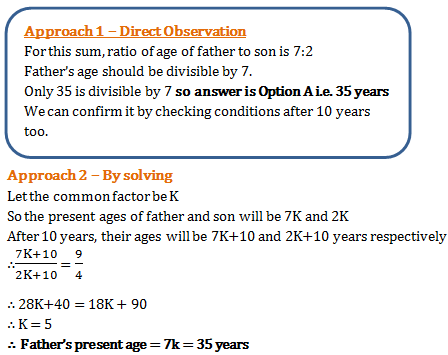4)   In a library, the ratio of number of story books to that of non-story books was 4:3 and total number of story books was 1248. When some more story books were bought, the ratio became 5:3. Find the number of story books bought.
- Published on 11 Apr 17

a. 312
b. 321
c. 936
d. 1560
 Answer  Explanation ANSWER: 312 Explanation: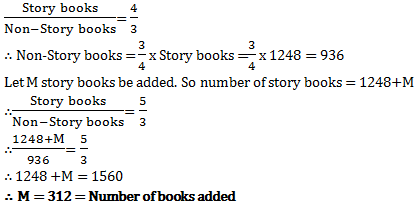5)   Ajay and Raj together have Rs. 1050. On taking Rs. 150 from Ajay, Ajay will have same amount as what Raj had earlier. Find the ratio of amounts with Ajay and Raj initially.
- Published on 11 Apr 17

a. 3:4
b. 7:1
c. 1:3
d. 4:3
 Answer  Explanation ANSWER: 4:3 Explanation: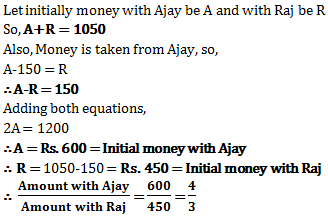6)   Price of each article of type P, Q, and R is Rs. 300, Rs. 180 and Rs. 120 respectively. Suresh buys articles of each type in the ratio 3:2:3 in Rs. 6480. How many articles of type Q did he purchase?
- Published on 11 Apr 17

a. 8
b. 14
c. 20
d. None of the above
 Answer  Explanation ANSWER: 8 Explanation: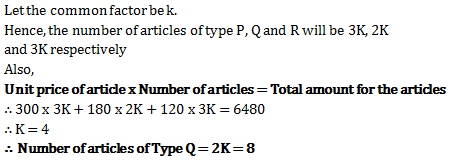7)   The ratio of market prices of wheat and paddy is 2:3 and the ratio of quantities consumed in a family is 5:4. Find the ratio of expenditure of wheat and paddy.
- Published on 07 Jul 17

a. 6:5
b. 5:6
c. 1:1
d. 8:15
 Answer  Explanation ANSWER: 5:6 Explanation: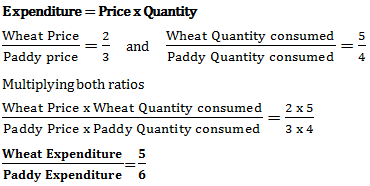8)   The ratio of numbers of girls and boys participating in sports of a school is 4:5. If the number of girls is 212, determine the number of boys participating in the sports.
- Published on 07 Jul 17

a. 256
b. 265
c. 251
d. 263
 Answer  Explanation ANSWER: 265 Explanation: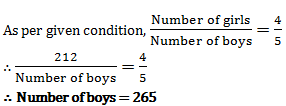9)   The three numbers are in the ratio 1/2 : 2/3 : 3/4. The difference between greatest and smallest numbers is 36. Find the numbers.
- Published on 07 Jul 17

a. 72, 84, 108
b. 60, 72, 96
c. 72, 84, 96
d. 72, 96, 108
 Answer  Explanation ANSWER: 72, 84, 108 Explanation: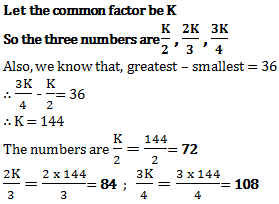10)   If A:B = 2:3, B:C = 4:5 and C:D = 6:7, then A:B:C:D is
- Published on 07 Jul 17

a. 18:24:30:35
b. 16:24:30:35
c. 16:22:30:35
d. 16:24:15:35
 Answer  Explanation ANSWER: 16:24:30:35 Explanation: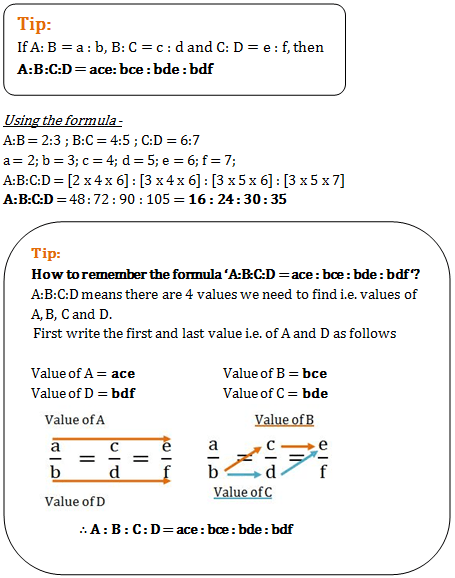1 2 3 4 5 6